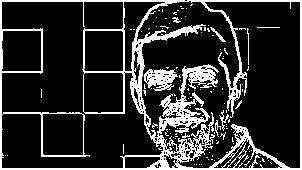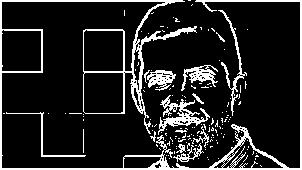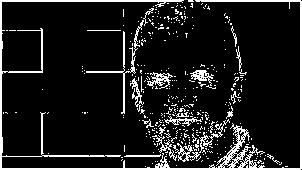# Constructing Edge Detectors from scratch in MATLAB...

by 4hathacker  |  in Image Processing at  10:48 PM

Hello everyone...!!!

I have posted about edge detection in one of the articles. But never have covered the whole thing from scratch. This post covers all of the edge detectors with introduction to convolution, filtering in images and pyramid function in MATLAB.

Edges, the sharp intensity variations present in the images help us to segment the objects, also the foreground and background. We know that, Images can be defined as a two dimensional function, f(x,y), where x and y are spatial coordinates, and the amplitude of f at any pair of coordinates (x,y) is called intensity of image at that point. Edges can be detected as meaningful discontinuities in intensity values using derivatives. The first order derivative is known as gradient of 2-D function f(x,y). While the second order derivatives are computed using Laplacian of f(x,y).

There are several approximations made to the derivatives for edge detection in images like Sobel, Prewitt, etc. The different edge detector masks are described in the code in matrix form for a typical neighborhood of 9 pixels.
The masks shown above slides over the image results in filtering to show for the edges. This is an example of discrete convolution taking place.The filtered values are given by linear combination of neighbourhood pixels, the specific weights are determined by mask/kernel values. The test image for this code is taken from Mathworks.Test Image
Let us see now, how for the edge detector script is defined in MATLAB. The default input set for the script is a grayscale image with a method for respective detector and a threshold value for basis of detection. Then, masks are created for horizontal and vertical detection. With each mask in two dimensions separately defined, convolution in 2-D is performed. The magnitude value in absolute terms is added and threshold is set for maximum value present after masking. This threshold is then used for whole image for detecting edges. The results so obtained are given below.

%%%%%%%%%%%%%%%%%%%%%%%%%%%%%%%%%%%%%%%%%%%%%%%%%%%%%%%%%%%%%%%%%%%%%%%%%%
%%%%% Name: Nitin Sharma
%%%%% Code: myedge.m
%%%%% MATLAB version: R2014a
%%%%% Toolbox Required: Image Processing Toolbox
%%%%% Utility: Understanding basics of edge detection, Convolution,
%%%%%          Filtering, Implementing different edge detectors
%%%%%%%%%%%%%%%%%%%%%%%%%%%%%%%%%%%%%%%%%%%%%%%%%%%%%%%%%%%%%%%%%%%%%%%%%
function detectedge = myedge(image,method,Threshold)

%%% Threshold must be greater than or equal to zero.
if Threshold < 0
error('Specify correct value of Threshold...');
return
end

%%% Creating different masks for edge detection
%%% Sobel Approximation
SobelX = [-1 0 1; -2 0 2; -1 0 1];
SobelY = [1 2 1; 0 0 0; -1 -2 -1];

%%% Roberts Approximation
RobertsX = [1 0; 0 -1];
RobertsY = [0 -1; 1 0];

%%% Prewitt Approximation
PrewittX = [-1 0 1; -1 0 1; -1 0 1];
PrewittY = [1 1 1; 0 0 0; -1 -1 -1];

%%% LoG Approximation
LoGXY = [1 1 1; 1 -8 1; 1 1 1];

switch lower(method)
case 'sobel'
Xdir = conv2(image, SobelX);
Ydir = conv2(image, SobelY);
Magnitude = abs(Xdir) + abs(Ydir);
Threshold = max(max(Magnitude))*Threshold;
Size = size(image);
for X = 1:Size(1),
for Y = 1:Size(2),
if Magnitude(X,Y) >= Threshold;
Magnitude(X,Y) = 255;
else
Magnitude(X,Y) = 0;
end
end
end
disp('Sobel edge detection.');
detectedge = Magnitude;

case 'roberts'
Xdir = conv2(image, RobertsX);
Ydir = conv2(image, RobertsY);
Magnitude = abs(Xdir) + abs(Ydir);
Threshold = max(max(Magnitude))*Threshold;
Size = size(image);
for X = 1:Size(1),
for Y = 1:Size(2),
if Magnitude(X,Y) >= Threshold;
Magnitude(X,Y) = 255;
else
Magnitude(X,Y) = 0;
end
end
end
disp('Roberts edge detection.');
detectedge = Magnitude;

case 'prewitt'
Xdir = conv2(image, PrewittX);
Ydir = conv2(image, PrewittY);
Magnitude = abs(Xdir) + abs(Ydir);
Threshold = max(max(Magnitude))*Threshold;
Size = size(image);
for X = 1:Size(1),
for Y = 1:Size(2),
if Magnitude(X,Y) >= Threshold;
Magnitude(X,Y) = 255;
else
Magnitude(X,Y) = 0;
end
end
end
disp('Prewitt edge detection.');
detectedge = Magnitude;

case 'log'
image = conv2(image, LoGXY);
Magnitude = abs(image);
Threshold = max(max(Magnitude))*Threshold;
Size = size(image);
for X = 1:Size(1),
for Y = 1:Size(2),
if Magnitude(X,Y) >= Threshold;
Magnitude(X,Y) = 255;
else
Magnitude(X,Y) = 0;
end
end
end
disp('LoG edge detection.');
detectedge = Magnitude;
end

The testing commands to be run on the command window are given below with the results obtained.

Sobel edge detection.
Prewitt edge detection.
LoG edge detection.
Roberts edge detection.Prewitt edge detectionSobel edge detectionRoberts edge detectionLoG edge detection

The results from LoG edge detection are not so good as compared to others. Sobel edge detection is fine. Prewitt has some inherent noise with results as compared to Sobel edge detection. Roberts edge detector is simplest and dominant for speedy manipulations of edges.